# How to use BigQuery aggregate functionsWritten by
Marcos Murata## How to use BigQuery aggregate functions

Analyzing large datasets to gain insights can be a daunting task. With terabytes or even petabytes of data, manually reviewing rows and columns is impossible. This is where BigQuery aggregate functions come in handy.

BigQuery is Google’s fully managed enterprise data warehouse that allows you to run fast queries on large datasets. One of the greatest benefits of BigQuery is its ability to aggregate data – combining information from multiple rows to help you gain insights.

With these functions, you can easily summarize and analyze large amounts of data.

In this article, you’ll learn everything you need to start using simple functions such as COUNT, SUM, AVG, MIN and MAX.

## What are aggregate functions in BigQuery?

Aggregate functions are an essential component of BigQuery. These powerful functions enable you to derive meaningful insights from large datasets by performing calculations across multiple rows of data.

With aggregate functions, you can combine multiple rows of data into a single result or output. Some examples include calculating totals, averages, maximums or minimums across a range of data.

Whether you need to sum revenue over time, find the average order value, or count the number of clients – aggregate functions have you covered. Here are some examples of these functions.

AVG: returns the average of all non-null values.

COUNT: returns the total number of rows.

COUNTIF: returns the number of rows that meet a specified condition.

MAX: returns the maximum non-null value.

MIN: returns the minimum non-null value.

SUM: returns the sum of all non-null values.

Let’s take a look at these aggregate functions and learn how to make the most of them. We’ll use this public dataset containing information about all the world population divided by country, from the year 1960 onwards.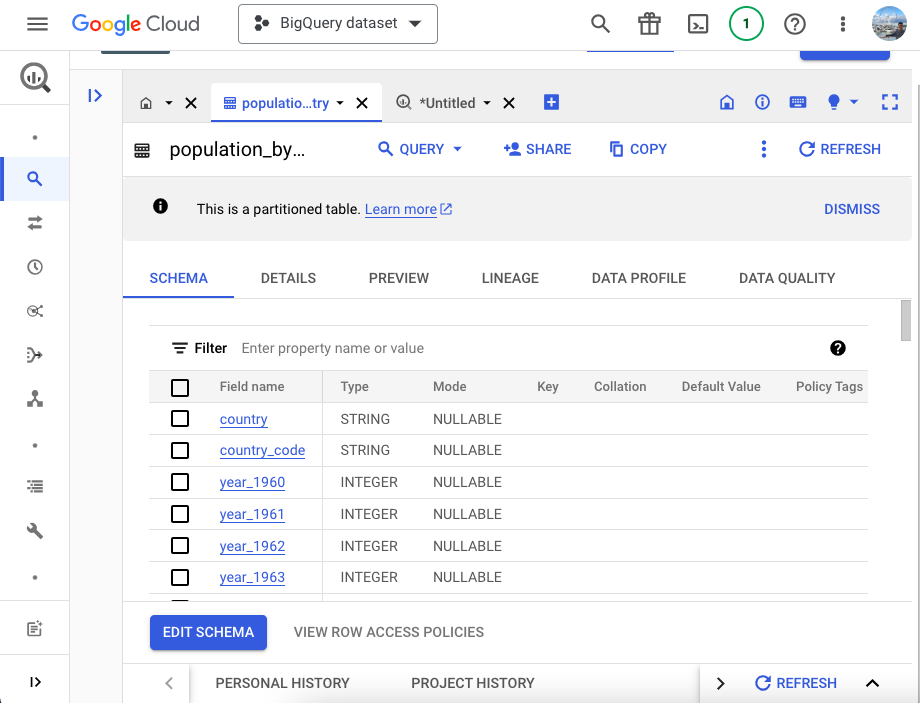## BigQuery aggregate functions: AVG

Let’s start with the AVG function. This function will return the average of all non-null values. Here’s the syntax:

SELECT AVG(column)

FROM table_name;

I’ll write a query to calculate the average of the population of these 6 countries in 2000: China, Germany, Russia, Japan, India, Brazil.

SELECT AVG(year_2000)

FROM `bigquery-public-data.world_bank_global_population.population_by_country`

WHERE country IN (‘China’, ‘Germany’, ‘Russia’, ‘Japan’, ‘India’, ‘Brazil’)

LIMIT 100;

Let’s break this formula down:

SELECT AVG(year_2000) – This statement specifies that we want to calculate the average of the specified column.

FROM `bigquery-public-data.world_bank_global_population.population_by_country` – This specifies the table we are querying.

WHERE country IN (‘China’, ‘Germany’, ‘Russia’, ‘Japan’, ‘India’, ‘Brazil’) – This WHERE clause filters the rows to only those countries in parentheses.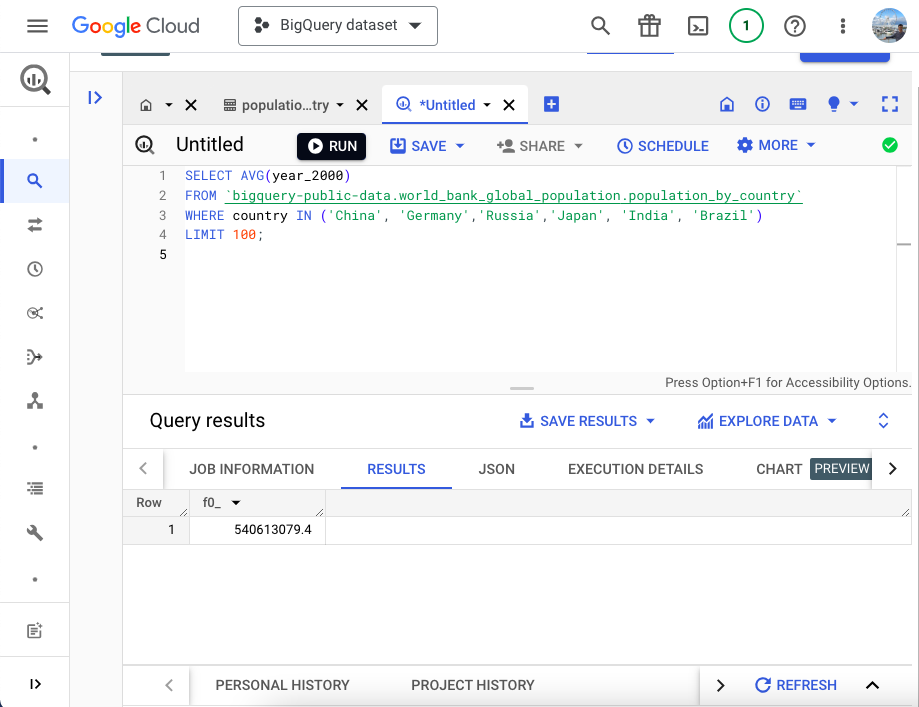When we run the query, BigQuery returns the average of the population of these 6 countries.

## BigQuery aggregate functions: COUNT

Now we’ll take a closer look at the COUNT function. When we run this function, BigQuery returns the total number of rows. Here’s the syntax we’ll use:

SELECT COUNT(column)

FROM table_name;

I’ll run a simple query to count the number of rows contained in the table. We’ll use the “country” column as a reference.

SELECT COUNT(country)

FROM `bigquery-public-data.world_bank_global_population.population_by_country`

LIMIT 100;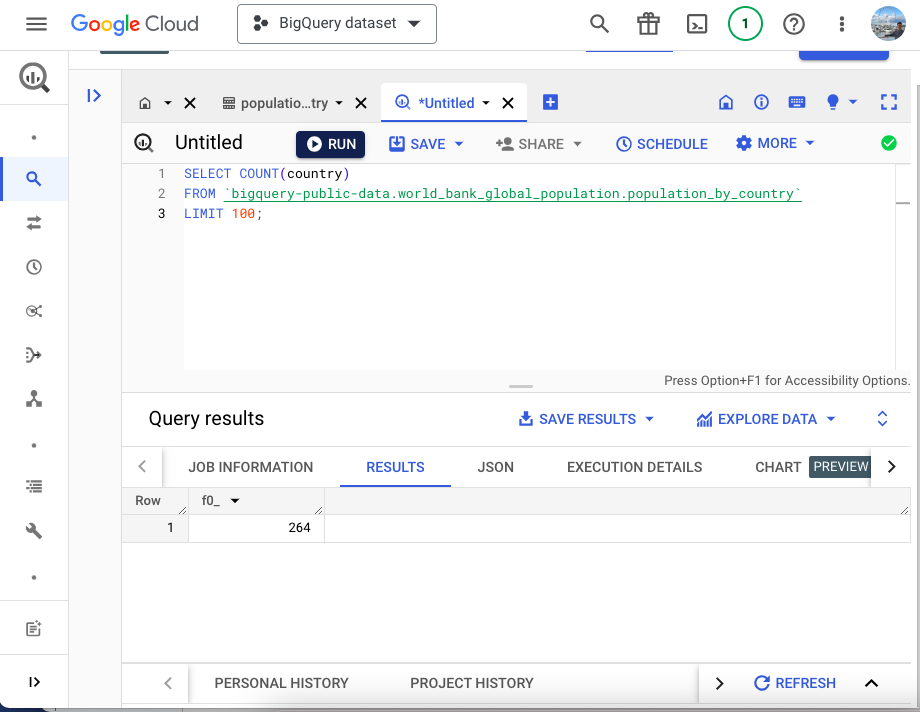After running this query, BigQuery has returned the number of rows: 264.

## BigQuery aggregate functions: COUNTIF

With the COUNTIF function, BigQuery will return the number of rows that meet a certain condition. Here’s the syntax:

SELECT COUNTIF(condition)

FROM table_name;

Let’s say I want BigQuery to return the number of rows where the population is less than 100.000 in a specific year. I’ll write the query specifying the condition: column year_2000 is less than 100.000.

This is what the query would look like:

SELECT COUNTIF(year_2000<100000)

FROM `bigquery-public-data.world_bank_global_population.population_by_country`

LIMIT 100;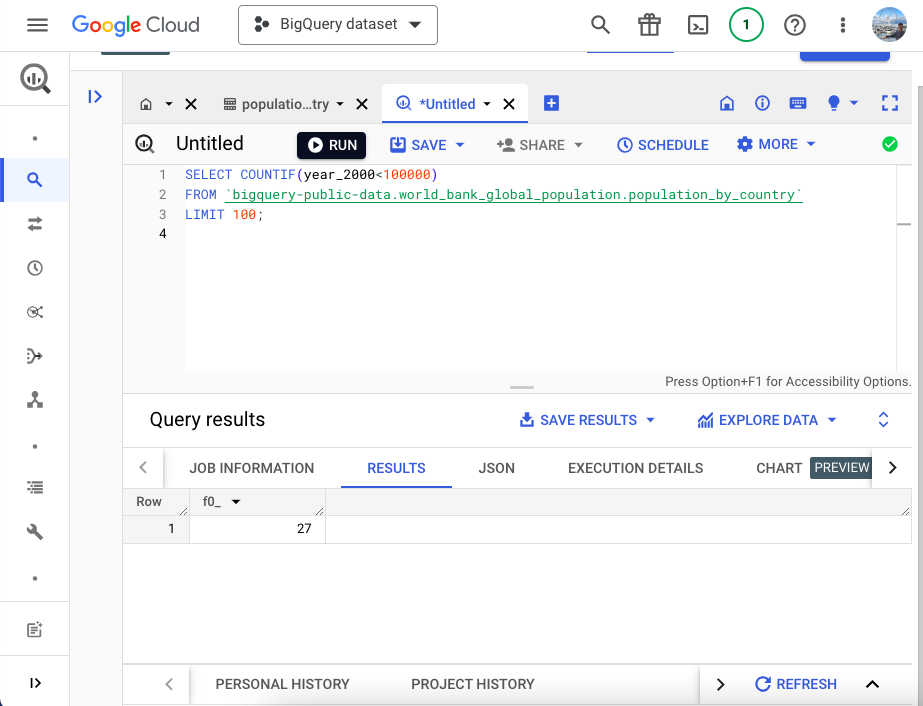When I execute this query, BigQuery returns the number of rows that meet this condition: 27.

## BigQuery aggregate functions: MAX

By using the MAX function, I can ask BigQuery to return the maximum non-null value in a specific column. This is the syntax we’ll use.

SELECT MAX(column)

FROM table_name;

I’ll write a query that will return the maximum value contained in the year_2000 column. In addition, I’ll add a WHERE clause to restrict the query to these 6 countries: China, Germany, Russia, Japan, India, Brazil.

SELECT MAX(year_2000)

FROM `bigquery-public-data.world_bank_global_population.population_by_country`

WHERE country IN (‘China’,’Germany’,’Russia’,’Japan’, ‘India’, ‘Brazil’)

LIMIT 100;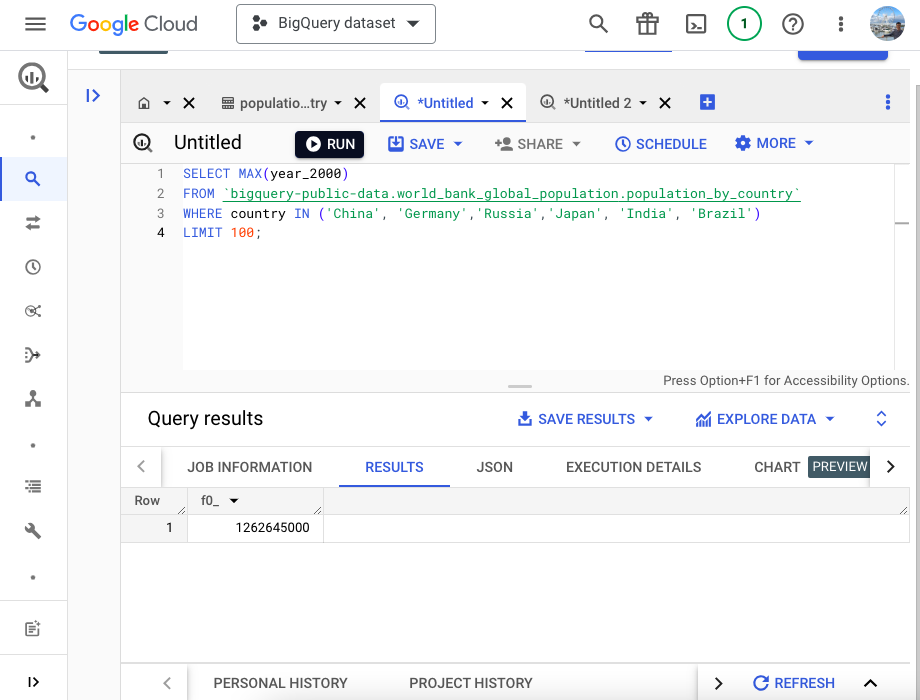When I execute the query, it returns the maximum value within the specified range: 1,262,645,000.

## BigQuery aggregate functions: MIN

As opposed to the MAX function, the MIN function will return the minimum value in a column. This is what the syntax looks like.

SELECT MIN(column)

FROM table_name;

Now, I’ll just replace the MAX function with MIN.

SELECT MIN(year_2000)

FROM `bigquery-public-data.world_bank_global_population.population_by_country`

WHERE country IN (‘China’, ‘Germany’,’Russia’,’Japan’, ‘India’, ‘Brazil’)

LIMIT 100;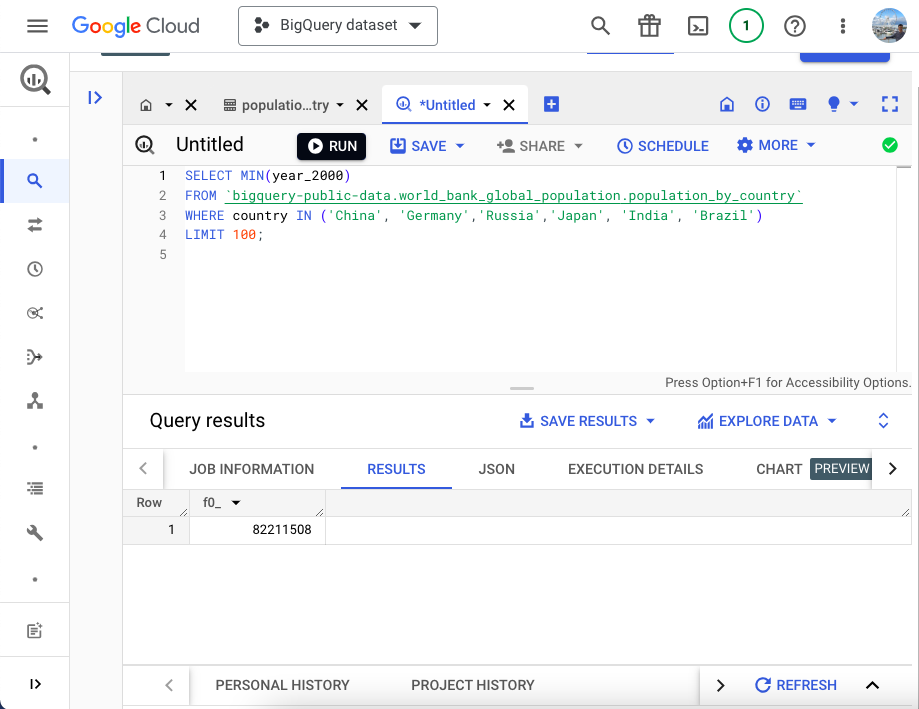BigQuery has just returned the minimum value. The smallest population is north of 82 million.

## BigQuery aggregate functions: SUM

Lastly, we’ll take a closer look at the SUM function. By using this function, I’ll ask BigQuery to calculate the sum of all the values.

SELECT SUM(column)

FROM table_name;

Here, I’ll write a query to calculate the sum of the population of the same 6 countries.

SELECT SUM(year_2000)

FROM `bigquery-public-data.world_bank_global_population.population_by_country`

WHERE country IN (‘China’, ‘Germany’,’Russia’,’Japan’, ‘India’, ‘Brazil’)

LIMIT 100;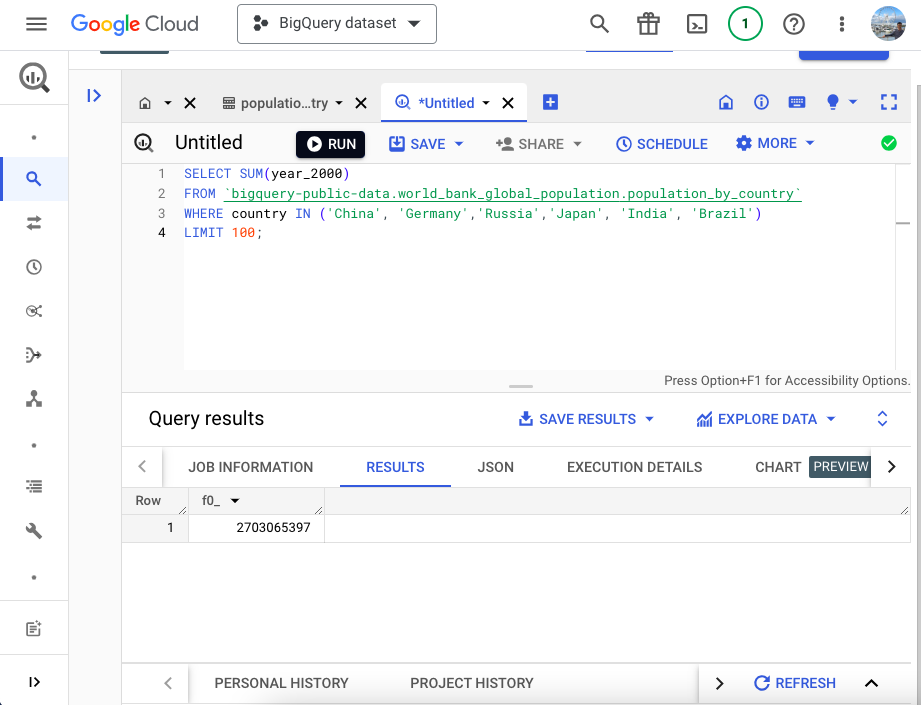As a result, BigQuery has calculated the population of these 6 countries: over 2.7 billion.

## How to use BigQuery aggregate functions

There you have it! That’s how you can use aggregate functions in BigQuery. By using these functions, you can combine and summarize data from multiple rows into a single value. If you want to combine tables instead, check out this article on how to use BigQuery joins.